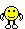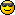<![CDATA[Math Is Fun Forum / what number?]]> 2007-10-25T10:50:20Z FluxBB https://www.mathisfunforum.com/viewtopic.php?id=8426 <![CDATA[Re: what number?]]> soha,
The post has been edited by Khushboo. She has replaced 14 by 21 in  post#2.]]>
https://www.mathisfunforum.com/profile.php?id=682 2007-10-25T10:50:20Z https://www.mathisfunforum.com/viewtopic.php?pid=81206#p81206
<![CDATA[Re: what number?]]> Khushboo wrote:

oops i am sorry

Jane is correct
it should be

=>x+2/3x=35
=>5x/3=35
or x=21

and not  14

Thanxs

i see 21 there, not 14

]]>
https://www.mathisfunforum.com/profile.php?id=4385 2007-10-25T07:49:15Z https://www.mathisfunforum.com/viewtopic.php?pid=81196#p81196
<![CDATA[Re: what number?]]> simplified in my language:

a)35 is (5/3) or 1 2/3 times of the number. Find 1 times the number.
b)the smaller number is 1/2(50-10). Just add 10 to that result and you have the greater number.

]]>
https://www.mathisfunforum.com/profile.php?id=7190 2007-10-25T05:38:07Z https://www.mathisfunforum.com/viewtopic.php?pid=81171#p81171
<![CDATA[Re: what number?]]> oops i am sorry

Jane is correct
it should be

=>x+2/3x=35
=>5x/3=35
or x=21

and not  14

Thanxs

]]>
https://www.mathisfunforum.com/profile.php?id=9708 2007-10-23T11:01:50Z https://www.mathisfunforum.com/viewtopic.php?pid=81024#p81024
<![CDATA[Re: what number?]]> Khushboo wrote:

Let the no. be x
=>x+2/3x=35
=>5x/3=35
or x=21

]]>
https://www.mathisfunforum.com/profile.php?id=6777 2007-10-23T10:23:57Z https://www.mathisfunforum.com/viewtopic.php?pid=81023#p81023
<![CDATA[Re: what number?]]> Let the no. be x
=>x+2/3x=35
=>5x/3=35
or x=21

b) let the no. be x,y
=> x+y=50......1)
=>x-y=10.........2)
you can simultaneously solve and cancel y
=> 2x=60
or x=30
similarly solve for y, cancel out x by putting minus sign beneath both the equations.
=>2y=40
or y=20

this should help

khushboo

]]>
https://www.mathisfunforum.com/profile.php?id=9708 2007-10-23T08:26:32Z https://www.mathisfunforum.com/viewtopic.php?pid=81020#p81020
<![CDATA[what number?]]> A)A number added to its  two thirds is equal to 35.Find the number.
B)The sum of two numbers is 50 , their diffrence is 10 .Find the numbers.

thanks:)

]]>
https://www.mathisfunforum.com/profile.php?id=4385 2007-10-23T06:38:04Z https://www.mathisfunforum.com/viewtopic.php?pid=81017#p81017